### IMO Shortlist 2007 problem A1

Kvaliteta:
Avg: 3,0
Težina:
Avg: 6,0
Real numbers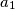$a_{1}$,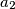$a_{2}$,$\ldots$,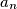$a_{n}$ are given. For each$i$,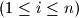$(1 \leq i \leq n )$, define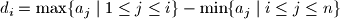and let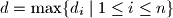$d = \max \{d_{i}\mid 1 \leq i \leq n \}$.

(a) Prove that, for any real numbers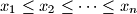$x_{1}\leq x_{2}\leq \cdots \leq x_{n}$,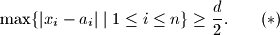(b) Show that there are real numbers$x_{1}\leq x_{2}\leq \cdots \leq x_{n}$ such that the equality holds in (*).

Author: Michael Albert, New Zealand
Izvor: Međunarodna matematička olimpijada, shortlist 2007